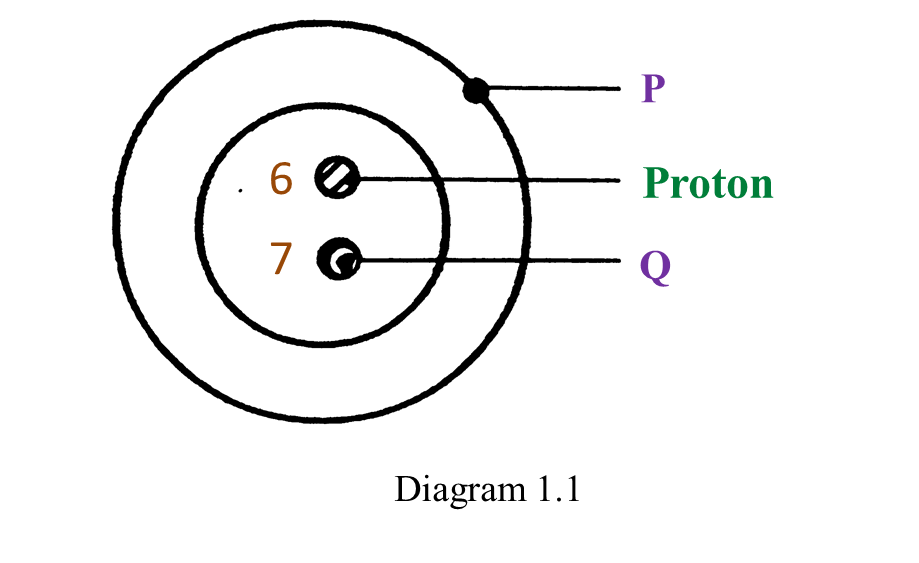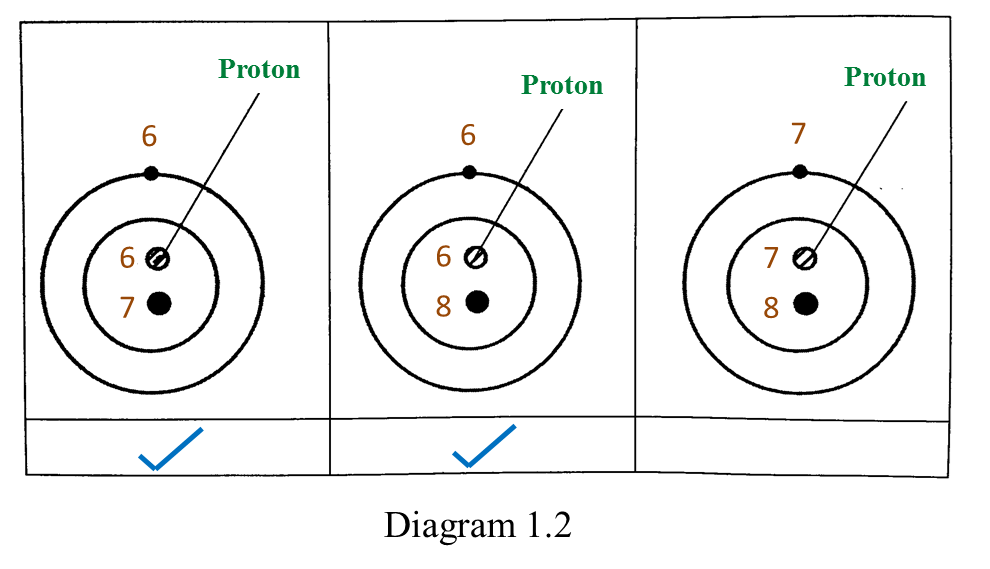# 4.9.3 Proton Number and Nucleon Number (Structured Questions)

Question 1:
Diagram 1.1 shows the structure of an atom.(a) P and Q are subatomic particles.
Name P and Q.
(b) What is the charge of P?
(c) What is the nucleon number of this atom?
(d) Diagram 1.2 shows the structure of three atoms.
(i) Which atoms are isotopes?(ii) Based on Diagram 1.2, complete the sentence below.
Isotopes are atoms of an element which have different number of nucleons but the same number of ______________.

(a)
P: Electron
Q: Neutron

(b)
Negative

(c)
13

(d)(i)(d)(ii)

Protons